撰文： Homer Pacag

:

Ransom:Win32/Tovicrypt.A (Microsoft)

平台：

Windows

整體風險評比: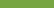潛在威脅性：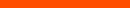潛在散播風險：報告感染 :資訊暴露評比：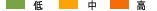•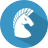惡意程式類型：
Trojan
•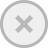具破壞性：
•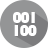被加密：
•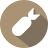In the wild:

## 總覽與描述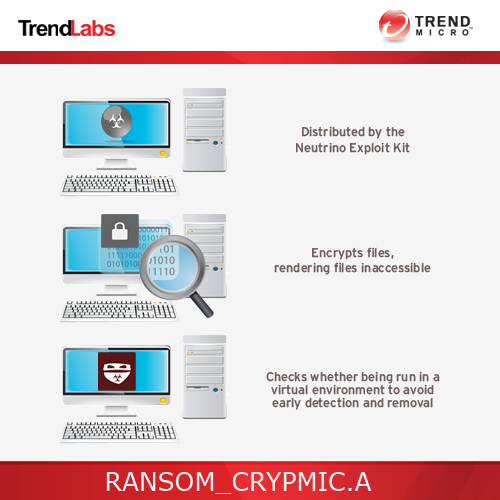## 詳細技術資訊

• %User Temp%\README.TXT - text ransom note
• %User Temp%\README.HTML - webpage ransom note
• %User Temp%\README.BMP - wallpaper/image ransom note
• %User Temp%\PAB.KEY
• {folders containing encrypted files}\README.HTML - ransom note
• {folders containing encrypted files}\README.BMP - ransom note
• {folders containing encrypted files}\README.TXT - ransom note

(注意：%User Temp% 是目前使用者的 Temp 資料夾，通常是 C:\Documents and Settings\{user name}\Local Settings\Temp（Windows 2000、XP 和 Server 2003）。)

• %User Startup%\README.TXT - text ransom note
• %User Startup%\README.HTML - webpage ransom note
• %User Startup%\README.BMP - image ransom note

(注意：%User Startup% 是目前使用者的「啟動」資料夾，通常是 C:\Windows\Profiles\{user name}\「開始」功能表\程式集\啟動（Windows 98 和 ME）、C:\WINNT\Profiles\{user name}\「開始」功能表\程式集\啟動 (Windows NT)，以及 C:\Documents and Settings\{User name}\「開始」功能表\程式集\啟動。)

• It encrypts files and retains original filename and extension

• .3DM
• .3DS
• .3G2
• .3GP
• .4DB
• .4DL
• .4MP
• .A3D
• .ABM
• .ABS
• .ABW
• .ACCDB
• .ACT
• .AES
• .AF2
• .AF3
• .AFT
• .AFX
• .AGIF
• .AGP
• .AHD
• .AIC
• .AIF
• .AIM
• .ALBM
• .ALF
• .ANI
• .ANS
• .APD
• .APK
• .APM
• .APNG
• .APP
• .APS
• .APT
• .APX
• .ARC
• .ART
• .ARW
• .ASC
• .ASE
• .ASF
• .ASM
• .ASP
• .ASPX
• .ASW
• .ASX
• .ASY
• .ATY
• .AVI
• .AWDB
• .AWP
• .AWT
• .AWW
• .AZZ
• .BAY
• .BBS
• .BDB
• .BDP
• .BDR
• .BEAN
• .BIB
• .BM2
• .BMP
• .BMX
• .BNA
• .BND
• .BOC
• .BOK
• .BRD
• .BRK
• .BRN
• .BRT
• .BSS
• .BTD
• .BTI
• .BTR
• .BZ2
• .C4D
• .CAL
• .CALS
• .CAN
• .CD5
• .CDB
• .CDC
• .CDG
• .CDMM
• .CDMT
• .CDR
• .CDR3
• .CDR4
• .CDR6
• .CDT
• .CER
• .CFG
• .CFM
• .CFU
• .CGI
• .CGM
• .CIMG
• .CIN
• .CIT
• .CKP
• .CLASS
• .CLKW
• .CMA
• .CMD
• .CMX
• .CNM
• .CNV
• .COLZ
• .CPC
• .CPD
• .CPG
• .CPP
• .CPS
• .CPT
• .CPX
• .CRD
• .CRT
• .CRWL
• .CRYPT
• .CSR
• .CSS
• .CSV
• .CSY
• .CUE
• .CV5
• .CVG
• .CVI
• .CVS
• .CVX
• .CWT
• .CXF
• .CYI
• .DAF
• .DB3
• .DBF
• .DBK
• .DBT
• .DBV
• .DBX
• .DCA
• .DCB
• .DCH
• .DCS
• .DCT
• .DCU
• .DCX
• .DDL
• .DDOC
• .DDS
• .DED
• .DF1
• .DGN
• .DGS
• .DHS
• .DIB
• .DIF
• .DIP
• .DIZ
• .DJV
• .DJVU
• .DM3
• .DMI
• .DMO
• .DNC
• .DNE
• .DOC
• .DOCB
• .DOCM
• .DOCX
• .DOCZ
• .DOT
• .DOTM
• .DOTX
• .DP1
• .DPP
• .DPX
• .DQY
• .DRW
• .DRZ
• .DSK
• .DSN
• .DSV
• .DT2
• .DTA
• .DTD
• .DTSX
• .DTW
• .DVI
• .DVL
• .DWG
• .DXB
• .DXF
• .DXL
• .ECO
• .ECW
• .ECX
• .EDB
• .EFD
• .EGC
• .EIO
• .EIP
• .EIT
• .EMD
• .EMF
• .EML
• .EMLX
• .EPF
• .EPP
• .EPS
• .EPSF
• .EQL
• .ERF
• .ERR
• .ETF
• .ETX
• .EUC
• .EXR
• .FAL
• .FAQ
• .FAX
• .FB2
• .FB3
• .FBL
• .FBX
• .FCD
• .FCF
• .FDB
• .FDF
• .FDR
• .FDS
• .FDT
• .FDX
• .FDXT
• .FES
• .FFT
• .FH10
• .FH11
• .FH3
• .FH4
• .FH5
• .FH6
• .FH7
• .FH8
• .FIC
• .FID
• .FIF
• .FIG
• .FIL
• .FLA
• .FLI
• .FLR
• .FLV
• .FM5
• .FMV
• .FODT
• .FOL
• .FP3
• .FP4
• .FP5
• .FP7
• .FPOS
• .FPT
• .FPX
• .FRM
• .FRT
• .FT10
• .FT11
• .FT7
• .FT8
• .FT9
• .FTN
• .FWDN
• .FXC
• .FXG
• .FZB
• .FZV
• .GBK
• .GBR
• .GCDP
• .GDB
• .GDOC
• .GED
• .GEM
• .GEO
• .GFB
• .GGR
• .GIF
• .GIH
• .GIM
• .GIO
• .GLOX
• .GPD
• .GPG
• .GPN
• .GPX
• .GRO
• .GROB
• .GRS
• .GSD
• .GTHR
• .GTP
• .GWI
• .HBK
• .HDB
• .HDP
• .HDR
• .HHT
• .HIS
• .HPG
• .HPGL
• .HPI
• .HPL
• .HTC
• .HTM
• .HTML
• .HWP
• .I3D
• .IBD
• .IBOOKS
• .ICN
• .ICON
• .IDC
• .IDEA
• .IDX
• .IFF
• .IGT
• .IGX
• .IHX
• .IIL
• .IIQ
• .IMD
• .INDD
• .INFO
• .INI
• .INI0
• .INI4
• .INI8
• .INID
• .INIH
• .INIL
• .INIP
• .INIT
• .INIX
• .INK
• .IPF
• .IPX
• .ITDB
• .ITW
• .IWI
• .J2C
• .J2K
• .JAR
• .JAS
• .JAVA
• .JB2
• .JBMP
• .JBR
• .JFIF
• .JIA
• .JIS
• .JKS
• .JNG
• .JOE
• .JP1
• .JP2
• .JPE
• .JPEG
• .JPG
• .JPG2
• .JPS
• .JPX
• .JRTF
• .JSP
• .JTX
• .JWL
• .JXR
• .KDB
• .KDBX
• .KDC
• .KDI
• .KDK
• .KES
• .KEY
• .KIC
• .KLG
• .KML
• .KMZ
• .KNT
• .KON
• .KPG
• .KWD
• .LAY
• .LAY6
• .LBM
• .LBT
• .LDF
• .LGC
• .LIS
• .LIT
• .LJP
• .LMK
• .LNT
• .LP2
• .LRC
• .LST
• .LTR
• .LTX
• .LUA
• .LUE
• .LUF
• .LWO
• .LWP
• .LWS
• .LYT
• .LYX
• .M3D
• .M3U
• .M4A
• .M4V
• .MAC
• .MAN
• .MAP
• .MAQ
• .MAT
• .MAX
• .MBM
• .MBOX
• .MDB
• .MDF
• .MDN
• .MDT
• .MEF
• .MELL
• .MFD
• .MFT
• .MGCB
• .MGMT
• .MGMX
• .MID
• .MIN
• .MKV
• .MMAT
• .MML
• .MNG
• .MNR
• .MNT
• .MOBI
• .MOS
• .MOV
• .MP3
• .MP4
• .MPA
• .MPF
• .MPG
• .MPO
• .MRG
• .MRXS
• .MS11
• .MSG
• .MSI
• .MT9
• .MUD
• .MWB
• .MWP
• .MXL
• .MYD
• .MYI
• .MYL
• .NCR
• .NCT
• .NDF
• .NEF
• .NFO
• .NJX
• .NLM
• .NOTE
• .NOW
• .NRW
• .NS2
• .NS3
• .NS4
• .NSF
• .NV2
• .NYF
• .NZB
• .OBJ
• .OC3
• .OC4
• .OC5
• .OCE
• .OCI
• .OCR
• .ODB
• .ODG
• .ODM
• .ODO
• .ODP
• .ODS
• .ODT
• .OFL
• .OFT
• .OMF
• .OPLC
• .OQY
• .ORA
• .ORF
• .ORT
• .ORX
• .OTA
• .OTG
• .OTI
• .OTP
• .OTS
• .OTT
• .OVP
• .OVR
• .OWC
• .OWG
• .OYX
• .OZB
• .OZJ
• .OZT
• .P12
• .P7S
• .P96
• .P97
• .PAGES
• .PAL
• .PAN
• .PANO
• .PAP
• .PAQ
• .PAS
• .PBM
• .PC1
• .PC2
• .PC3
• .PCD
• .PCS
• .PCT
• .PCX
• .PDB
• .PDD
• .PDF
• .PDM
• .PDN
• .PDS
• .PDT
• .PE4
• .PEF
• .PEM
• .PFF
• .PFI
• .PFS
• .PFV
• .PFX
• .PGF
• .PGM
• .PHM
• .PHP
• .PI1
• .PI2
• .PI3
• .PIC
• .PICT
• .PIF
• .PIX
• .PJPG
• .PJT
• .PLT
• .PLUGIN
• .PMG
• .PNG
• .PNI
• .PNM
• .PNTG
• .PNZ
• .POP
• .POT
• .POTM
• .POTX
• .PP4
• .PP5
• .PPAM
• .PPM
• .PPS
• .PPSM
• .PPSX
• .PPT
• .PPTM
• .PPTX
• .PRF
• .PRIV
• .PRIVATE
• .PRT
• .PRW
• .PSD
• .PSDX
• .PSE
• .PSID
• .PSP
• .PSPIMAGE
• .PSW
• .PTG
• .PTH
• .PTX
• .PVJ
• .PVM
• .PVR
• .PWA
• .PWI
• .PWR
• .PXR
• .PZ3
• .PZA
• .PZP
• .PZS
• .QCOW2
• .QDL
• .QMG
• .QPX
• .QRY
• .QVD
• .RAR
• .RAS
• .RAW
• .RCTD
• .RCU
• .RDB
• .RDDS
• .RDL
• .RFT
• .RGB
• .RGF
• .RIB
• .RIC
• .RIFF
• .RIS
• .RIX
• .RLE
• .RLI
• .RNG
• .RPD
• .RPF
• .RPT
• .RRI
• .RSB
• .RSD
• .RSR
• .RST
• .RTD
• .RTF
• .RTX
• .RUN
• .RW2
• .RWL
• .RZK
• .RZN
• .S2MV
• .S3M
• .SAF
• .SAI
• .SAM
• .SAVE
• .SBF
• .SCC
• .SCH
• .SCI
• .SCM
• .SCT
• .SCV
• .SCW
• .SDB
• .SDF
• .SDM
• .SDOC
• .SDW
• .SEP
• .SFC
• .SFW
• .SGM
• .SIG
• .SITX
• .SK1
• .SK2
• .SKM
• .SLA
• .SLD
• .SLDX
• .SLK
• .SLN
• .SLS
• .SMF
• .SMIL
• .SMS
• .SOB
• .SPA
• .SPE
• .SPH
• .SPJ
• .SPP
• .SPQ
• .SPR
• .SQB
• .SQL
• .SQLITE3
• .SQLITEDB
• .SR2
• .SRT
• .SRW
• .SSA
• .SSK
• .STC
• .STD
• .STE
• .STI
• .STM
• .STN
• .STP
• .STR
• .STW
• .STY
• .SUB
• .SUMO
• .SVA
• .SVF
• .SVG
• .SVGZ
• .SWF
• .SXC
• .SXD
• .SXG
• .SXI
• .SXM
• .SXW
• .T2B
• .TAB
• .TAR
• .TB0
• .TBK
• .TBN
• .TCX
• .TDF
• .TDT
• .TEX
• .TEXT
• .TFC
• .TG4
• .TGA
• .TGZ
• .THM
• .THP
• .TIF
• .TIFF
• .TJP
• .TLB
• .TLC
• .TM2
• .TMD
• .TMP
• .TMV
• .TMX
• .TNE
• .TPC
• .TPI
• .TRM
• .TVJ
• .TXT
• .U3D
• .U3I
• .UDB
• .UFO
• .UFR
• .UGA
• .UNX
• .UOF
• .UOP
• .UOT
• .UPD
• .USR
• .UTF8
• .UTXT
• .V12
• .VBR
• .VBS
• .VCF
• .VCT
• .VCXPROJ
• .VDA
• .VDB
• .VDI
• .VEC
• .VFF
• .VMDK
• .VML
• .VMX
• .VNT
• .VOB
• .VPD
• .VPE
• .VRML
• .VRP
• .VSD
• .VSDM
• .VSDX
• .VSM
• .VST
• .VSTX
• .VUE
• .WAV
• .WB1
• .WBC
• .WBD
• .WBK
• .WBM
• .WBMP
• .WBZ
• .WCF
• .WDB
• .WDP
• .WEBP
• .WGZ
• .WIRE
• .WKS
• .WMA
• .WMDB
• .WMF
• .WMV
• .WP4
• .WP5
• .WP6
• .WP7
• .WPA
• .WPD
• .WPE
• .WPG
• .WPL
• .WPS
• .WPT
• .WPW
• .WRI
• .WSC
• .WSD
• .WSF
• .WSH
• .WTX
• .WVL
• .X3D
• .X3F
• .XAR
• .XCODEPROJ
• .XDB
• .XDL
• .XHTM
• .XHTML
• .XLC
• .XLD
• .XLF
• .XLGC
• .XLM
• .XLR
• .XLS
• .XLSB
• .XLSM
• .XLSX
• .XLT
• .XLTM
• .XLTX
• .XLW
• .XML
• .XPM
• .XPS
• .XWP
• .XY3
• .XYP
• .XYW
• .YAL
• .YBK
• .YML
• .YSP
• .YUV
• .Z3D
• .ZABW
• .ZDB
• .ZDC
• .ZIF
• .ZIP
• .ZIPX
• .ZZ

## 解決方案

VSAPI OPR 病毒碼版本: 12.637.00
VSAPI OPR 病毒碼發行日期： 2016年7月7日

Step 1

Step 3

[ 學到更多 ]

Step 4

[ 學到更多 ]

• In HKEY_CURRENT_USER\Control Panel\Desktop

Step 5

[ 學到更多 ]

• %User Temp%\PAB.KEY